Next: Name for Up: What is Previous: What is

## Why is ?

In modern mathematics, the string of symbols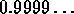is understood to be a shorthand for ``the infinite sum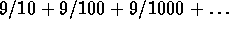''. This in turn is shorthand for ``the limit of the sequence of real numbers 9/10, 9/10 + 9/100,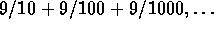''. Using the well-known epsilon-delta definition of the limit (you can find it in any of the given references on analysis), one can easily show that this limit is 1. The statement that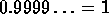is simply an abbreviation of this fact.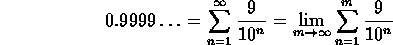Choose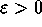. Suppose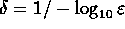, thus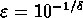. For every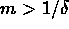we have that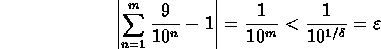So by the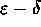definition of the limit we have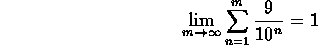Not formal enough? In that case you need to go back to the construction of the number system. After you have constructed the reals (Cauchy sequences are well suited for this case, see [Shapiro75]), you can indeed verify that the preceding proof correctly shows.

An informal argument could be given by noticing that the following sequence of ``natural'' operations has as a consequence. Therefore it's ``natural'' to assume.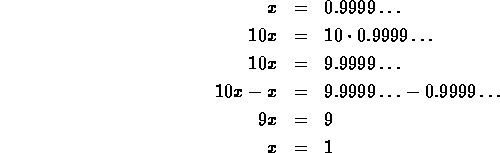Thus.

An even easier argument multiplies both sides of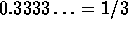by 3. The result is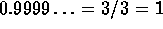.

Another informal argument is to notice that all periodic numbers such as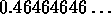are equal to the period divided over the same number of 9s. Thus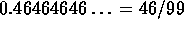. Applying the same argument to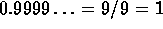.

Although the three informal arguments might convince you that, they are not complete proofs. Basically, you need to prove that each step on the way is allowed and is correct. They are also ``clumsy'' ways to prove the equality since they go around the bush: provingdirectly is much easier.

You can even have that while you are proving it the ``clumsy'' way, you get proof of the result in another way. For instance, in the first argument the first step is showing thatis real indeed. You can do this by giving the formal proof stated in the beginning of this FAQ question. But then you haveas corollary. So the rest of the argument is irrelevant: you already proved what you wanted to prove.

References

R.V. Churchill and J.W. Brown. Complex Variables and Applications.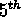ed., McGraw-Hill, 1990.

E. Hewitt and K. Stromberg. Real and Abstract Analysis. Springer-Verlag, Berlin, 1965.

W. Rudin. Principles of Mathematical Analysis. McGraw-Hill, 1976.

L. Shapiro. Introduction to Abstract Algebra. McGraw-Hill, 1975.Next: Name for Up: What is Previous: What is

Alex Lopez-Ortiz
Mon Feb 23 16:26:48 EST 1998